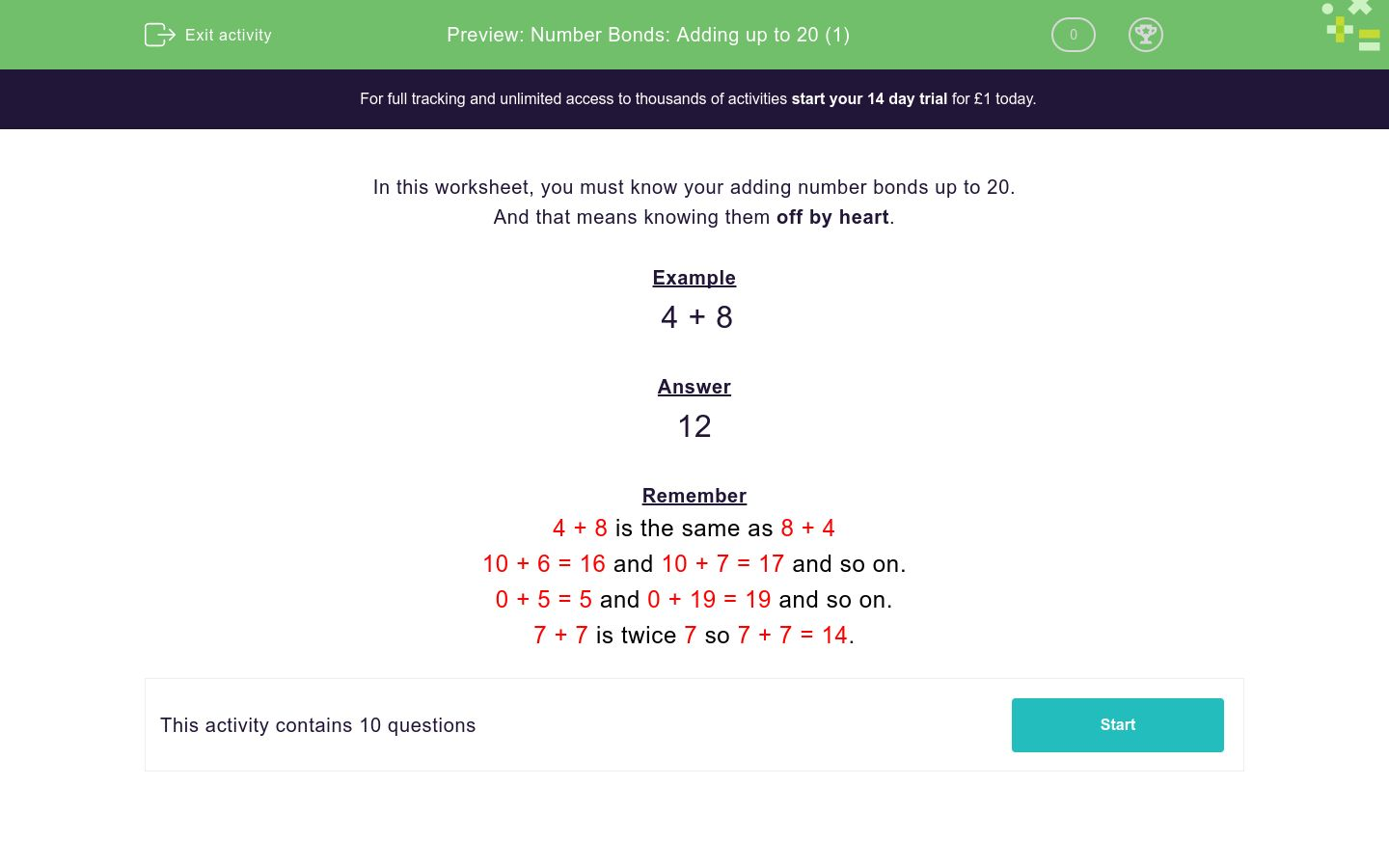# Number Bonds: Adding up to 20 (1)

In this worksheet, students must simply know their addition number bonds up to 20. No explanations are given.Key stage:  KS 1

Curriculum topic:  Number: Addition and Subtraction

Curriculum subtopic:  Add and Subtract to 20

Difficulty level:### QUESTION 1 of 10

In this worksheet, you must know your adding number bonds up to 20.

And that means knowing them off by heart.

Example

4 + 8

12

Remember

4 + 8 is the same as 8 + 4

10 + 6 = 16 and 10 + 7 = 17 and so on.

0 + 5 = 5 and 0 + 19 = 19 and so on.

7 + 7 is twice 7 so 7 + 7 = 14.

3 + 5

5 + 3

5 + 5

6 + 6

3 + 0

13 + 5

3 + 9

9 + 5

3 + 7

12 + 5

• Question 1

3 + 5

8
• Question 2

5 + 3

8
• Question 3

5 + 5

10
• Question 4

6 + 6

12
• Question 5

3 + 0

3
• Question 6

13 + 5

18
• Question 7

3 + 9

12
• Question 8

9 + 5

14
• Question 9

3 + 7

10
• Question 10

12 + 5

17
---- OR ----

Sign up for a £1 trial so you can track and measure your child's progress on this activity.

### What is EdPlace?

We're your National Curriculum aligned online education content provider helping each child succeed in English, maths and science from year 1 to GCSE. With an EdPlace account you’ll be able to track and measure progress, helping each child achieve their best. We build confidence and attainment by personalising each child’s learning at a level that suits them.

Get startedStart your £1 trial today.
Subscribe from £10/month.